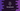# How to print a full pyramid in C++## How to print a full pyramid in C++ :

In this post, we will learn how to print a full pyramid in C++. The program will take the height of the pyramid as input from the user and print it out.

A full pyramid looks as like below:

``````    *
* *
* * *
* * * *
* * * * *``````

The height of this pyramid is 5. Our program will print the pyramid of any height.

### Algorithm to print a pyramid:

To print a pyramid, we need to find out how it is printed actually. Let’s take a look at the below pyramid:

``````yyyy*
yyy* *
yy* * *
y* * * *
* * * * *``````

This is almost similar to the above one. The only difference is that we are using y and the above pyramid uses blank space.

To learn how to print this triangle, let’s write the algo for the second triangle:

For this triangle, height is 5 and if we consider the row is starting from 0,

• For row 0, we are printing 4 y and 1 *.
• For row 1, we are printing 3 y and 2 *.
• For row 2, we are printing 2 y and 3 *.

So, for row n, we are printing height - n - 1 number of y and n + 1 number of *.

For the first triangle, we need to replace y with blank and add one space after each star.

So, the final algorithm will be:

• Take the height as input from the user
• Run one loop height number of times.
• Use two inner loops to print the blank and *. Print blank for height - i - 1 times and * for i + 1 times, where i is the current row as indicated by the loop.
• Add one blank after each * and don’t add any space between spaces.
• Add one new line after each row.

### C++ program:

Let’s write the above algorithm in C++ :

``````#include <iostream>
using namespace std;

int main()
{
int height;
cout << "Enter the height of the triangle : " << endl;
cin >> height;

for (int i = 0; i < height; i++)
{
for (int j = 0; j < (height - i - 1); j++)
{
cout << " ";
}
for (int j = 0; j < (i + 1); j++)
{
cout << "* ";
}
cout << endl;
}
}``````

Here,

• The for loop with i is used to run from i = 0 to i = height - 1.
• The inner for loops are used to print the blank spaces and *.
• The first loop prints from j = 0 to j = height - i - 2 a space.
• The second loop prints * from j = 0 to j = i.

### Output:

This program prints the below output:

``````Enter the height of the triangle :
10
*
* *
* * *
* * * *
* * * * *
* * * * * *
* * * * * * *
* * * * * * * *
* * * * * * * * *
* * * * * * * * * *

Enter the height of the triangle :
5
*
* *
* * *
* * * *
* * * * * ``````